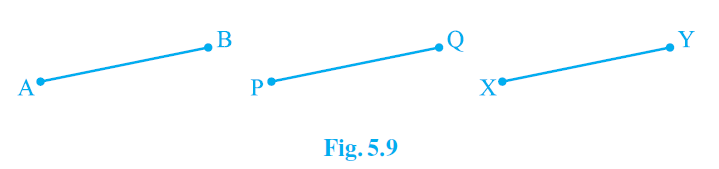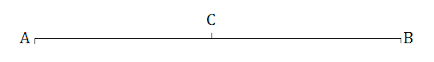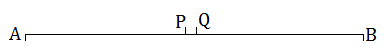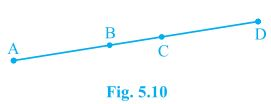# NCERT Solutions For Class 9 Math Chapter – 5 Exercise – 5.1

NCERT Solutions For Class 9 Math Chapter – 5 Exercise – 5.1

#### Experts at Sunstarup  have created the NCERT Solutions after extensive search on each topic Students can refer to this study study material to boost their confidence and attempt the second term exam smartly. The concepts are explained with steps, shortcuts to remember formula , tips and tricks to solve the numerical problems wisely and quickly.

Q1.

Which of the following statements are true and which are false ? Give reasons for your answers.

• Only one line can pass through a single point.
• There are an infinite number of lines which pass through two distinct points.
• A terminated line can be produced indefinitely on both sides.
• If two circles are equal , then their radii are equal.
• In Fig  If AB = PQ and PQ = XY then AB = XY.Solution:-

• There can be infinite line drawn passing through  a single point.
•  Only one line can be drawn which passes through two distinct points.
• A terminated line can be produced indefinitely on both sides In geometry A line can be extended in both direction. A line means infinite long length.
• If two circles are equal , then their  radii are equal.

By suspension we will find that the centre ad circumference of the both circles coincide. Hence , their radius must be equal.

• By Euclid first axiom things which are equal to the same things are equal to one another.

Q2. Give a definition for each of the following terms. Are there other terms that need to be defined first? What are they and how might you define them ?

• Parallel lines        (ii) Perpendicular lines       (iii) Line segment      (iv) Radius of a circle    (v) Square

Solution:-

Yes, other terms need to be a defined first which are:

Plane: A plane is flat surface on which geometric figures are drawn.

Point: A point is a dot drawn on a plane surface and is dimensionless.

Line: A line is collection of points which can extends in both direction and has only length not breadth.

Parallel lines: When two or more never interest  each other other in a  plane and perpendicular distance between them is always constant then they are said to be parallel lines.

Perpendicular lines: When two lines intersect each other at right angle in a plane then they are said to be perpendicular to each other.

Line segment: A line segment is a part of a line with two ends points and cannot be extended further.

Radius of circle: The fixed distance between the center and the circumference o the circle is called the radius of circle.

Square: A square is a quadrilateral in which all four sides are equal and each internal angle is a right angle.

Q3. Consider two ‘Postulates’ given below:

• Given any two distinct points A and B there exists third point C which is in between A and B.
• There exist at least three points that  are not on the same line.

Do these postulates contain any undefined terms? Are there postulates consistent ? Do they follow from Euclid postulates ? Explain.

Solution:-

Undefined terms in the postulates:

Many points lie in a plane But here is not given about the position of the point C whether it lies on the line segment joining AB or not.

Also , there is no information about the plane whether the points are in same plane or not.

Yes , these postulates are consistent when we deal with these two situation:

• Point C is lying in between and on the line segment joining A and B.
• Point C not lies on the line segment joining A and B.

Q4. If a point C lies between two points points A and B such that AC = BC then prove that AC = 1/2 AB. Explain by drawing the figure.

Solution:-Here AC = BC

AC + AC = BC + AC

Also , BC + AC = AB (As it coincides with line segment AB)

2AC = AB (If equals are added to equals the wholes are equal.)

AC = 1/2 AB.Q5. In Question 4. Point C is called a mid – point of line segment AB. Prove that every line segment has one and only one mid – point.

Solution:-Let A and B be the line segment and points P and Q be two different mid points of AB.

Now ,

P and Q are midpoints of AB.

Therefore AP  = PB  and also AQ = QB.

Also , PB + AP = AB (as its coincides with line segment AB)

Similarly QB + AQ = AB.

Now ,

AP + AP = PB + AP (If equals are added to equals , the wholes are equal.)

2AP – AB (i)

Similarly

2AQ = AB  (ii)

From (I) and (ii)

2AP = 2AQ (Things which are equal to the same thing are equal to one another.)

AP = PQ (Things which are doubled of the same things are equal to one another.)

Thus P and Q are the same point This contradicts the fact that P and Q are two different mid points of AB. Thus , it is proved hat every line segment has one and only one mid – point.

Q6. In Fig 5.10 If AC = BD then prove that AB = CD.Solution:-

Given , AC = BD

From the figure,

AC = AB + BC

BD = BC + CD

AB + BC = BC + BD

According to Euclid axiom when equal are subtracted from equal , remainders are also equal.

Subtracting BC both sides,

AB + BC – BC = BC + CD – BC

AB = CD

Q7. Why is axiom 5 , in the list Euclid axioms , considered a universal truth?

Solution:-

Axiom 5: The whole is always greater than the part .

Take an example of a cake. When it is whole it will measure 2 pound but when we took out a part from it and measures its weight it will come out lower than the previous one. So , the fifth axiom of Euclid is true for all the universal things That is  why it considered a ‘universal truth’.